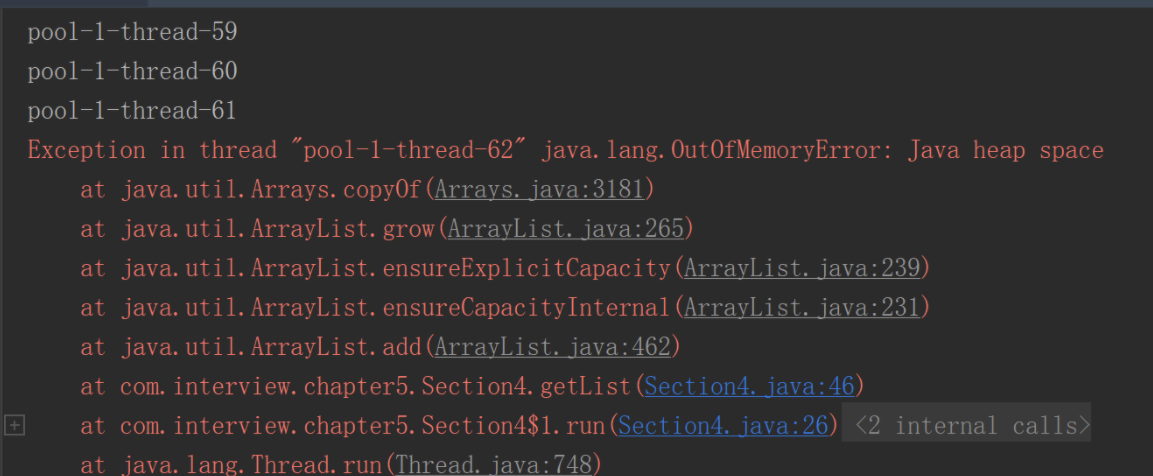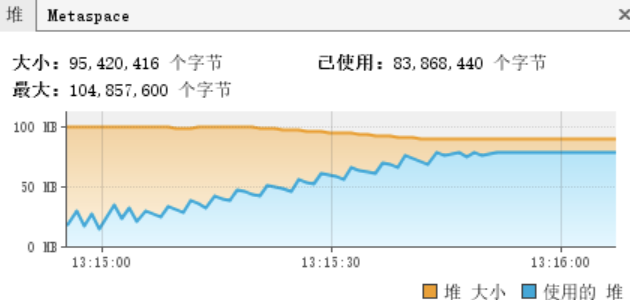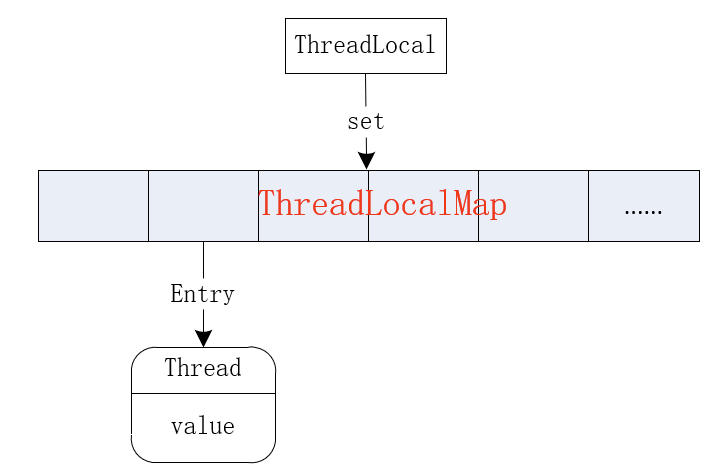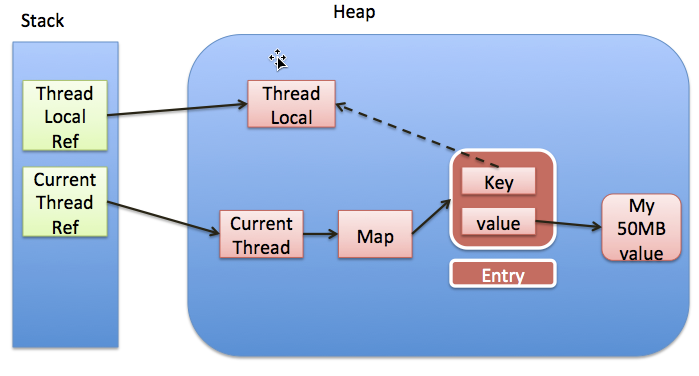``````ThreadLocal threadLocal = new ThreadLocal();
// 存值
// 取值
System.out.println(list.size());
//删除值

``````
2

[老宋, Java 面试题]

null
````````````ThreadLocal inheritableThreadLocal = new InheritableThreadLocal();

``老宋``

``````class ThreadLocalTest {
static Integer MOCK_MAX = 10000;
public static void main(String[] args) throws InterruptedException {
for (int i = 0; i < THREAD_MAX; i++) {
executorService.execute(() -> {
});
try {
} catch (InterruptedException e) {
e.printStackTrace();
}
}
executorService.shutdown();
}
List getList() {
List list = new ArrayList();
for (int i = 0; i < MOCK_MAX; i++) {
}
return list;
}
}``````## 内存溢出原理分析

``````public void set(T value) {
if (map != null)
map.set(this, value);
else
createMap(t, value);
}``````

``````private void set(ThreadLocal<?> key, Object value) {
Entry[] tab = table;
int len = tab.length;
int i = key.threadLocalHashCode & (len-1);
for (Entry e = tab[i];
e != null;
e = tab[i = nextIndex(i, len)]) {
if (k == key) {
e.value = value;
return;
}
if (k == null) {
replaceStaleEntry(key, value, i);
return;
}
}
tab[i] = new Entry(key, value);
int sz = ++size;
if (!cleanSomeSlots(i, sz) && sz >= threshold)
rehash();
}````````````class ThreadLocalTest {
static Integer MOCK_MAX = 10000;
public static void main(String[] args) throws InterruptedException {
for (int i = 0; i < THREAD_MAX; i++) {
executorService.execute(() -> {
// 移除对象
});
try {
} catch (InterruptedException e) {
e.printStackTrace();
}
}
executorService.shutdown();
}
List getList() {
List list = new ArrayList();
for (int i = 0; i < MOCK_MAX; i++) {